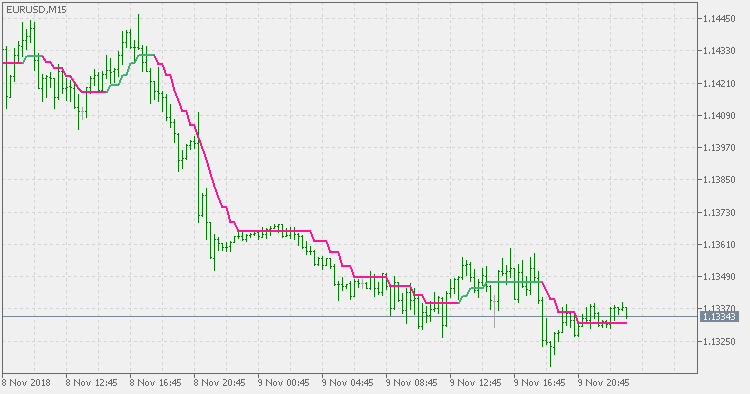Interesting script?
So post a link to it -
let others appraise it

You liked the script? Try it in the MetaTrader 5 terminal# Filtered averages - indicator for MetaTrader 5

Views:
1429
Rating:
Published:
2018.11.10 19:49
Updated:
2019.01.29 16:23

Theory:

The indicator that is using self adjusting filter (published and described here : Filtered Price ) but applied to one of the 4 types of averages :

• simple moving average (SMA)
• exponential moving average (EMA)
• smoothed moving average (SMMA)
• linear weighted moving average (LWMA)

Filter size is the "size" of self adjusted deviations and can be fractional.

Usage:

You can use the color change as a signal.Filtered price

Filtered price using self adjusting deviation for price filteringTriangular moving average

Triangular moving averageFiltered WPR

Filtered WPR (Williams Percent Range)Range weighted average

Range weighted average - average that uuse the current volatility for weighted average calculation